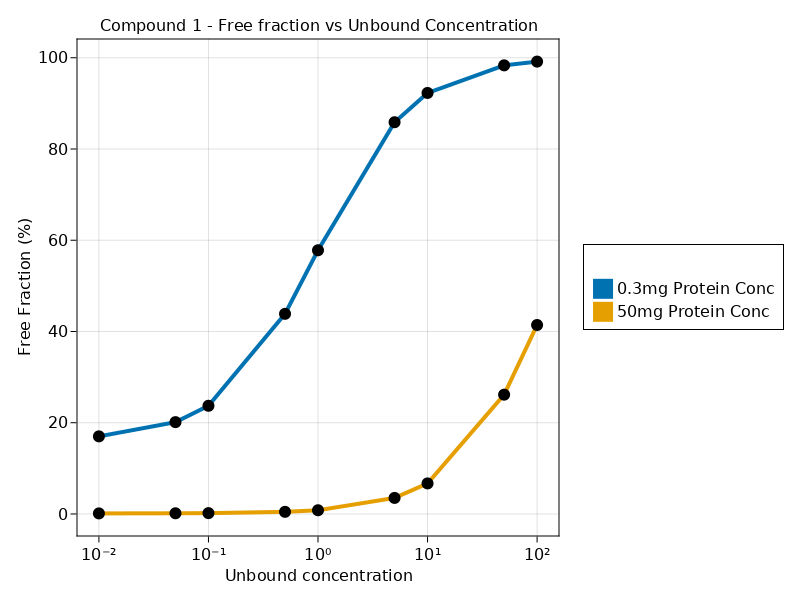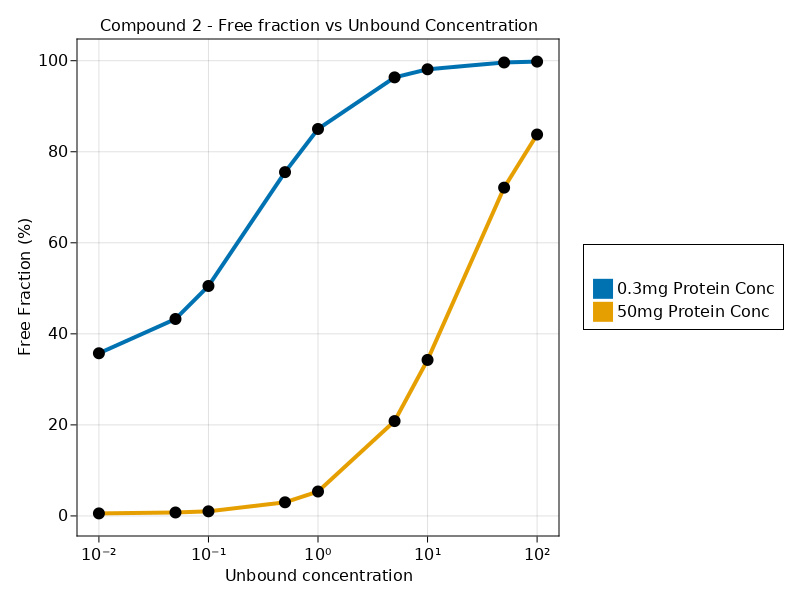# Exercise PK47 - Plasma Protein Binding Modeling

### Background

• Structural Model - Plasma Protein Binding Model

• Number of Subjects - 4

• Number of Compounds - 2

### Learning Outcome

• To get an understanding of the determinants of the unbound concentration, free fraction and total concentration.

• How the modeling of plasma protein data is done

• To understand the relationship how the binding protein concentration effects the unbound drug concentration

### Objectives

• To analyze In vitro plasma protein data of two compounds

• To analyze binding data at two different binding concentration

• To show and understand the relationship between Cu and fu

• To understand the properties of binding site that can be modeled

### Libraries

call the necessary 'libraries' to get started

using Random
using Pumas
using PumasUtilities
using CairoMakie
using AlgebraOfGraphics
using DataFramesMeta


### Model

pk_47     = @model begin
desc = "Plasma Protein Binding Model"
end

@param begin
"Affinity constant between drug and protein"
tvka  ∈ RealDomain(lower=0)
"Number of binding sites per molecule"
tvn   ∈ RealDomain(lower=0)
Ω     ∈ PDiagDomain(2)
end

@random begin
η     ~ MvNormal(Ω)
end

@covariates dose Pt

@pre begin
Ka    = tvka * exp(η)
n     = tvn * exp(η)
_dose = dose
_Pt   = Pt
end

@vars begin
fu    = (1-(1/(1+(_dose/(n*_Pt))+(1/(Ka*n*_Pt)))))*100
end

@derived begin
end
end

PumasModel
Random effects: η
Covariates: dose, Pt
Dynamical variables:
Derived: dv, fu
Observed: dv, fu


### Parameters

The parameters are as given below. tv represents the typical value for parameters.

• $tvka$ - Affinity constant between drug and protein

• $tvn$ - Number of binding sites per molecule

## Compound 1
param1 = (tvka  = 6.09,
tvn   = 2.833,
Ω     = Diagonal([0.0,0.0,0.0]),

## Compound 2
param2 = (tvka  = 10.2353,
tvn   = 1.937,
Ω     = Diagonal([0.0,0.0,0.0]),


### Dosage Regimen

Since there is no dosage regimen we will create a dataframe with no events and read the file using read_pumas which will be used for simulation.

## Compound 1
df_sub1  = DataFrame(id="0.3mg Protein Conc", time=0:1:9999, dv=missing, dose=0.01:0.01:100, Pt=0.3)
df_sub2  = DataFrame(id="50mg Protein Conc", time=0:1:9999, dv=missing, dose=0.01:0.01:100, Pt=50)
df1      = vcat(df_sub1, df_sub2)
pop1     = read_pumas(df1, observations=[:dv], covariates=[:dose, :Pt], event_data=false)

## Compound 2
df_sub3  = DataFrame(id="0.3mg Protein Conc", time=0:1:9999, dv=missing, dose=0.01:0.01:100, Pt=0.1)
df_sub4  = DataFrame(id="50mg Protein Conc", time=0:1:9999, dv=missing, dose=0.01:0.01:100, Pt=10)
df2      = vcat(df_sub3, df_sub4)
pop2     = read_pumas(df2, observations=[:dv], covariates=[:dose, :Pt], event_data=false)


### Simulation

We will now simulate the experimental dat for Compound 1 at two different levels of protein concentration(Pt) 0.3 and 50.

##Compound 1
Random.seed!(123)
sim_1    = simobs(pk_47, pop1, param1)
df47_cmp1 = DataFrame(sim_1)

## Compound 2
Random.seed!(123)
sim_2    = simobs(pk_47, pop2, param2)
df47_cmp2 = DataFrame(sim_2)


### Visualization

Compound 1

@chain df47_cmp1 begin
@rsubset :dose ∈ [0.01,0.05,0.1,0.5,1,5,10,50,100]
data(_) *
mapping(:dose => "Unbound concentration", :fu => "Free Fraction (%)", color = :id => "") *
visual(ScatterLines, linewidth = 4, markersize = 12)
draw(axis = (; yticks = 0:20:120, xscale = log10,
title = "Compound 1 - Free fraction vs Unbound Concentration",))
end@chain df47_cmp2 begin
@rsubset :dose ∈ [0.01,0.05,0.1,0.5,1,5,10,50,100]
data(_) *
mapping(:dose => "Unbound concentration", :fu => "Free Fraction (%)", color = :id => "") *
visual(ScatterLines, linewidth = 4, markersize = 12)
draw(axis = (; yticks = 0:20:120, xscale = log10,
title = "Compound 2 - Free fraction vs Unbound Concentration",))
end